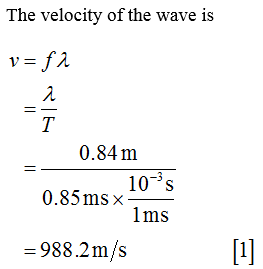# A piano string is under a tension of T = 872 N. When struck the wave has a period of t = 0.85 ms and a wavelength of λ = 0.84 m.T = 872 Nt = 0.85 msλ = 0.84 mWhat is the linear density of the string, in kilograms per meter?

Question
17 views

A piano string is under a tension of T = 872 N. When struck the wave has a period of t = 0.85 ms and a wavelength of λ = 0.84 m.

T = 872 N
t = 0.85 ms
λ = 0.84 m

What is the linear density of the string, in kilograms per meter?

check_circle

Step 1...

### Want to see the full answer?

See Solution

#### Want to see this answer and more?

Solutions are written by subject experts who are available 24/7. Questions are typically answered within 1 hour.*

See Solution
*Response times may vary by subject and question.
Tagged in